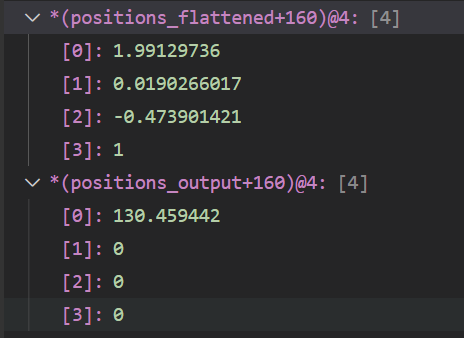# Trouble with CUBLAS GEMM Strided Batch

I’m trying to multiply many vectors (1x4) by many matrices (4x4) using a CUBLAS Gem operation.

I have them all in col-maj order (pretty sure - but I think it’s actually irrelevant to the bug here), in one contiguous memory allocation. So as far as I can see a Gemm strided batch (single point prec.) operation is perfect for what I’m trying to achieve.

I’ve double checked all of my parameters but I’m getting really strange results. If I write out a sample 1x4 and 4x4 matrix and calculate it by hand, the answer comes out as expected, but CUDA fills it with strange results.

The code I am using (I have renamed some variables for clarity - There are no syntax/variable name issues otherwise and it compiles and runs, the RaiseCuda functions simply check the output of each function):
int batches = 1000;

``````int floatsPerVector = 4;

int floatsPerMatrix = 16;

float *positions_output = new float[batches * floatsPerVector];

float *dev_mat_points = 0;

float *dev_mat_transform = 0;

float *dev_mat_result = 0;

int count_mat_points = batches * floatsPerVector;

int count_mat_transform = batches * floatsPerMatrix;

// reserve mem

RaiseCuda(cudaMalloc((void**)&dev_mat_points, count_mat_points * sizeof(float)));

RaiseCuda(cudaMalloc((void**)&dev_mat_transform, count_mat_transform * sizeof(float)));

RaiseCuda(cudaMalloc((void**)&dev_mat_result, count_mat_points * sizeof(float)));

// copy in data

RaiseCuda(cudaMemcpy(dev_mat_points, positions_flattened, count_mat_points * sizeof(float), cudaMemcpyHostToDevice));

RaiseCuda(cudaMemcpy(dev_mat_transform, tfs_flattened, count_mat_transform * sizeof(float), cudaMemcpyHostToDevice));

float alpha = 1.f;

float beta = 0.f;

HandleCublasStatus(cublasSgemmStridedBatched(cublasHandle, CUBLAS_OP_N, CUBLAS_OP_N,

1 /*m*/, 4 /*n*/, 4 /*k*/, &alpha,

dev_mat_transform /**B*/, floatsPerVector /**ldb*/, floatsPerTf /**strideB*/,

dev_mat_points /**A*/, floatsPerVector /*lda*/, floatsPerVector /*strideA*/,

&beta,

dev_mat_result /**C*/, floatsPerVector /**ldc*/, floatsPerVector /**strideC*/,

batches /**batchCount*/)

);

// wait for the result

// copy result back to host

RaiseCuda(cudaMemcpy(positions_output, dev_mat_result, count_mat_points * sizeof(float), cudaMemcpyDeviceToHost));

// clean up memory code here
``````

However these are the types of results I am getting which are unexpected, when I check a random Vector’s result:As far as I can see they may all follow the same format [N, 0, 0, 0] - Is this indicative of some sort of user error in copying back/interpreting the output? If I work out that matrix multiplication by hand it does not come out to those answers.

RUNNING ON: Nvidia jetson agx xavier
Cuda info:
Built on Wed_Oct_23_21:14:42_PDT_2019
Cuda compilation tools, release 10.2, V10.2.89

If you want to provide a complete test case, showing the actual output, as well as what you expect, I’ll take a look as time permits. I also recommend indicating CUDA version and the GPU you are running on.

Thanks Robert, the actual data is quite large (65k matrix operations), but I will boil it down to a reproducible sample.

Was just seeing if I was doing something obviously wrong with the parameters it could be picked up easily.

Using Cuda v10 on the Xavier

Cheers and will get back to you

EDITED: I believe it was as simple as having the m, n, k values not appropriately set. Will confirm but I’m pretty sure that was my issue. Thanks.,